# IBPS RRB 2018 Prelims : Quants – Day 9

Dear Bankersdaily Aspirants,

IBPS RRB PO & Office Assistant 2018 Preliminary Examination starts from 11th August, 2018. The IBPS RRB Exam 2018 is one of the most expected exam of the year. The reason for the consummate expectation is different from that of the others.

The number of candidates attending the IBPS RRB PO & Office Assistant is high when compared to the other exams like SBI & Insurance Exams. The other important factor is that , no English section is there in the preliminary examination of the IBPS RRB PO & Assistant Exams. This is really a boost to the candidates as many of the aspirants couldn’t get the requisite marks in the English section and this exam is the best bet for the aspirants.

Since only few days are left for the IBPS RRB PO & Assistant Exam 2018 Preliminary Exam , we will be providing daily 20 Questions in Quantitative Aptitude & Reasoning Section to evoke the preparation mode.

Aspirants can answer the Questions by commenting the answers for the Questions in the comments section. We will be providing the answers for the Questions @ 6:00 P.M everyday.

## IBPS RRB PO & Assistant 2018 – Prelims Exam – Day 9

Topic : Quantitative Aptitude

Total Number of Questions : 20

Total Time : 15 minutes

D.1–5) Find out the approximate value of X

Q.1)

39.977 + 64.99 x 2.001 – 35.121 x (1/7.009) = X

a) 165

b) 130

c) 175

d) 170

e) 135

Q.2)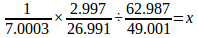a)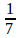b)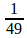c)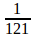d)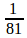e)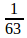Q.3) 19.99% of 520 + 25.010% of 700 – 15.979% of 300 = x

a) 252

b) 231

c) 263

d) 251

e) 255

Q.4)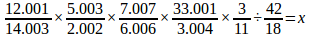a) 14/15

b) 15/43

c) 48/7

d) 6/7

e) 45/14

Q.5)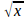+ 370% of 20 = 25% of 320

a) 36

b) 25

c) 49

d) 81

e) 64

D.6 -10) Find the missing number from the following series.

Q.6) 1, 17, 42, 78, 127,?

a) 181

b) 161

c) 191

d) 131

e) 121

Q.7) 1, 9, 36, 100, 225,?

a) 400

b) 324

c) 356

d) 484

e) 441

Q.8) 31, 41, 56, 78, 109, 151, ?

a) 200

b) 206

c) 212

d) 198

e) 194

Q.9) 120, 60, 90, 225, 787.5, ?

a) 3545.75

b) 3543.625

c) 3542.5

d) 3543.75

e) 3541.25

Q.10) 65, 66, 134, 405, 1624, ?

a) 8125

b) 8035

c) 8030

d) 8040

e) 8145

Q.11) M and N can do a work in 30 days, O can do the same work in 60 days, M is 50% more efficient than O. In how many days N alone can complete the work?

a) 120 days

b) 60 days

c) 70 days

d) 45 days

e) 54 days

Q.12) The ratio of girls and boys in a class out of 66 students is 4 : 7, and the boys and girls coming to the class from the village side are in the ratio of 3 : 4, then the non-villagers boys in the class is 27. Find how many non-village girls are there in the class?

a) 2

b) 4

c) 6

d) 8

e) Cannot be determined

Q.13) The value of X is 120% of Y. And the value Y is 20% less than the value of Z, then the value of the difference between the X and Z is 70. Find the value of X + Y + Z.

a) 4710

b) 4830

c) 4650

d) 4920

e) 4540

Q.14) A rectangle shape plate rod has the length and breadth of 12 meters and 9 metres respectively, will be melted and form as a circular shape. A cylinder has put on the circular rod, and the cylinder is exactly fitted to the circular rod. The height of the cylinder is 8 metres. Then, find the volume of the cylinder.

a) 256

b) 372

c) 336

d) 332

e) 338

Q.15) An average age of 70 passengers in a bus is 45, then the average age of first 30 passengers is 47 and the last 35 passengers is 40, then find out the average age of the remaining passengers in the bus?

a) 62

b) 58

c) 54

d) 60

e) 68

Q.16) The total age of father and mother is 6 times of the total age of a son and daughter, and the ratio of ages of son and daughter is 4 : 3, the son is 3 years elder than the daughter. Then, find the age difference between the father and mother.

a) 6 years

b) 3 years

c) 12 years

d) 24 years

e) Cannot be determined.

Q.17) Two trains X and Y are moving towards each other in parallel tracks. Both of the trains X and Y are moving at the speed of 80km/h and 100km/h, respectively. Both the trains meet after 5 hours. What is time taken by train Y to reach X’s starting point after they were meet?

a) 5 hours

b) 4 hours

c) 6 hours

d) 3 hours

e) 4.5 hours

Q.18) The difference between the simple and compound interest at the rate of 7% for 2 years is equal to 70% of 490 on a same sum, then find the sum.

a) 70000

b) 60000

c) 14000

d) 16000

d) 15000

Q.19) The cost price of X is equal to the selling price of Y and the cost price of Y is 400 less than the selling price of X, and the profit of X is 20%. Find the profit percentage of Y.

a) 20%

b) 15%

c) 10%

d) 25%

e) Cannot be determined.

Q.20) Two buckets M and N contain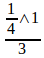of water respectively, and the remaining are milk. The ratio of the volume of two buckets is 4 : 5. Both the buckets are poured into a barrel. The resultant mixture contains 57 litres of milk. Find the volume of bucket N?

a) 30 litres

b) 35 litres

c) 40 litres

d) 45 litres

e) 50 litres

Explanations

Q.1) a

= 40 + 130 – 5

= 165.

Q.2) d

=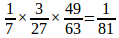Q.3) b

20% of 520 = 104

25% of 700 = 175

16% of 300 = 48

Then, 104 + 175 – 48 is = 279 – 48 = 231.

Q.4) e

=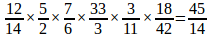Q.5) a

25% of 320 = 80

20% of 370 = 74

80 – 74 = 6

6 =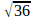Q.6) c

Differences = 16, 25, 36, 49

In this series, the next value = 64

Then 64 + 127 is = 191.

Q.7) e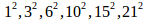Difference between the base value = 1, 2, 3, 4, 5, 6….

Q.8) b

Difference = 10, 15, 22, 31, 42…

Difference of difference = 5, 7, 9, 11, 13

42 + 13 = 55

Then, 55 + 151 is = 206.

Q.9) d

The series is =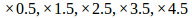787.5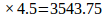Q.10) a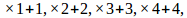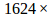5 +5 = 8120 + 5 = 8125

Q.11) a

Work efficiency of M and O = 1.5 : 1

Then, the number of days required by M and O = 1 : 1.5

M alone can do the work =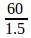= 40 days.

M and N do the work in 30 days.

N alone can do the work =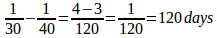Q.12) b

Girls and boys = 4 : 7 = 24 : 42

Then, the number of village boys = 42 – 27 = 15

The ratio of village boys to village girls = 3 : 4 = 15 : 20

Then, the number of non-village girls = 24 – 20 = 4.

Q.13) b

Ratio of X, Y and Z

X Y Z

6 5

4 5

————————-

24 20 25

————————-

Difference between X and Z = 25 – 24 = 70

Then X + Y + Z = 24 + 20 + 25 = 69

Then total =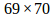= 4830.

Q.14) c

Perimeter of a rectangle = Circumference of a Circle.

Perimeter of the rectangle = 2(l+b) = 2 (12 + 9) = 42

Volume of a Cylinder = 2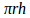2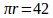2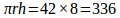Q.15) e

Total age =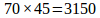Age of first 30 =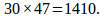Age of last 35 =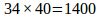Remaining age = 3150 – (1410 + 1400) = 340

Average age of remaining passengers =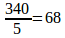Q.16) e

By the given information, we get only the total age of Father and Mother. We don’t know about their individual ages. So, we could not find the answer.

Q.17) b

=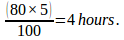Q.18) a

70% of 490 is = 343.

Difference between Si and Ci = 343

=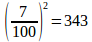Then 100% =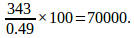Q.19) d

X Y

CP 1000 800

SP 1200 1000

Required percentage =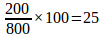Q.20) d

M N

WATER 9 15

Milk 27 30

————————-

36 45

—————————

We will post this Practice Questions – Daily till the start of the IBPS RRB PO & Assistant (Regularly)

Quantitative Aptitude @ 3:00 P.M

Reasoning Ability -@ 5:00 P.M

 QUANTITATIVE APTITUDE REASONING DAY 1 DAY 1 DAY 2 DAY 2 DAY 3 DAY 3 DAY 4 DAY 4 DAY 5 DAY 5 DAY 6 DAY 6 DAY 7 DAY 7 DAY 8 DAY 8

Attend GEEK TEST – SOLVE 10 Daily and enhance your preparation to the next level. Attend the Daily Tests with the Timer and improve your solving ability.

Also Please attend this BLUE WHALE QUESTION (which is a combination of many topics and combined into a single one). This is a One Stop Solution for all your Miscellaneous needs.

## BLUE WHALE CHALLENGE QUESTIONS

Get the FULL LENGTH MOCK TEST SERIES for SBI PO Mains 2018 , SBI CLERK Mains 2018 & BOB PO Mains 2018 from our Bankersdaily Store.